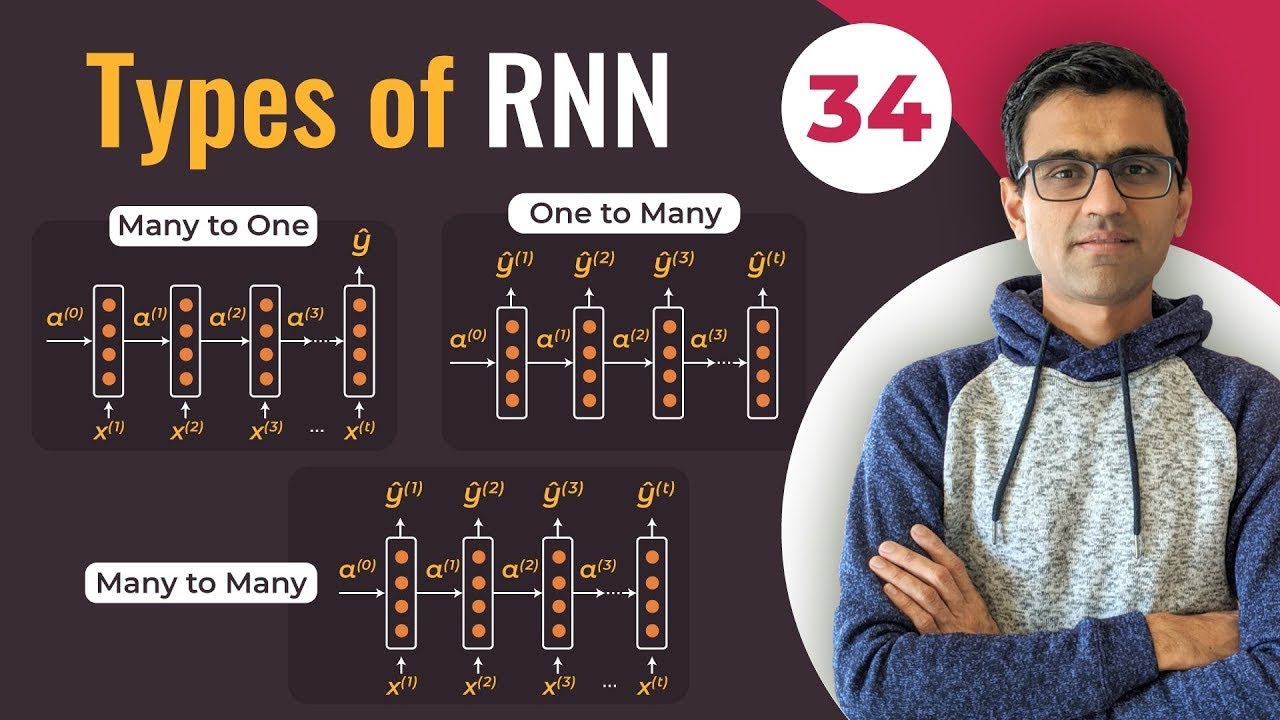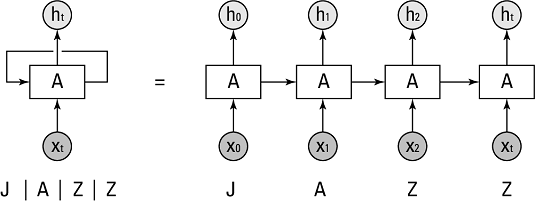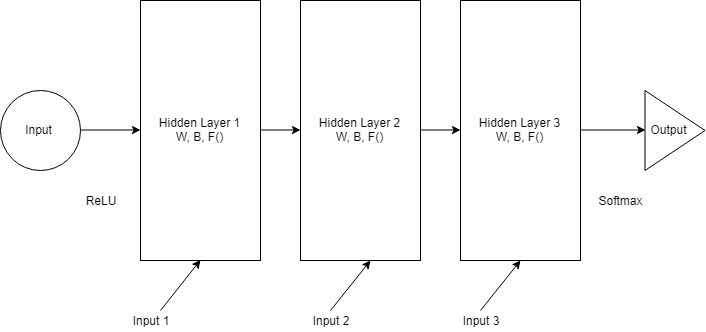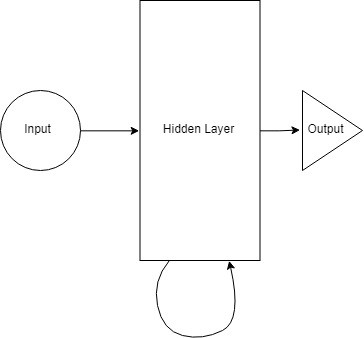1610720500

# Types of RNN | Recurrent Neural Network Types | Deep Learning Tutorial 34 (TensorFlow & Python)

Types of RNN | Recurrent Neural Network Types | Deep Learning Tutorial 34 (TensorFlow & Python)

In this video we will discuss different types of RNN types such as,

1. One to many
2. Many to many
3. Many to one

#deep-learning #python #tensorflow

## Buddha Community1619518440

## top 30 Python Tips and Tricks for Beginners

Welcome to my Blog , In this article, you are going to learn the top 10 python tips and tricks.

### 8) Check The Memory Usage Of An Object.

#python #python hacks tricks #python learning tips #python programming tricks #python tips #python tips and tricks #python tips and tricks advanced #python tips and tricks for beginners #python tips tricks and techniques #python tutorial #tips and tricks in python #tips to learn python #top 30 python tips and tricks for beginners1610720500

## Types of RNN | Recurrent Neural Network Types | Deep Learning Tutorial 34 (TensorFlow & Python)

Types of RNN | Recurrent Neural Network Types | Deep Learning Tutorial 34 (TensorFlow & Python)

In this video we will discuss different types of RNN types such as,

1. One to many
2. Many to many
3. Many to one

#deep-learning #python #tensorflow1593156510

## Basic Data Types in Python | Python Web Development For Beginners

At the end of 2019, Python is one of the fastest-growing programming languages. More than 10% of developers have opted for Python development.

In the programming world, Data types play an important role. Each Variable is stored in different data types and responsible for various functions. Python had two different objects, and They are mutable and immutable objects.

I Mutable objects

II Immutable objects

III Built-in data types in Python

## Mutable objects

The Size and declared value and its sequence of the object can able to be modified called mutable objects.

Mutable Data Types are list, dict, set, byte array

## Immutable objects

The Size and declared value and its sequence of the object can able to be modified.

Immutable data types are int, float, complex, String, tuples, bytes, and frozen sets.

id() and type() is used to know the Identity and data type of the object

a**=25+**85j

type**(a)**

output**:<class’complex’>**

b**={1:10,2:“Pinky”****}**

id**(b)**

output**:**238989244168

## Built-in data types in Python

a**=str(“Hello python world”)****#str**

b**=int(18)****#int**

c**=float(20482.5)****#float**

d**=complex(5+85j)****#complex**

e**=list((“python”,“fast”,“growing”,“in”,2018))****#list**

f**=tuple((“python”,“easy”,“learning”))****#tuple**

g**=range(10)****#range**

h**=dict(name=“Vidu”,age=36)****#dict**

i**=set((“python”,“fast”,“growing”,“in”,2018))****#set**

j**=frozenset((“python”,“fast”,“growing”,“in”,2018))****#frozenset**

k**=bool(18)****#bool**

l**=bytes(8)****#bytes**

m**=bytearray(8)****#bytearray**

n**=memoryview(bytes(18))****#memoryview**

## Numbers (int,Float,Complex)

Numbers are stored in numeric Types. when a number is assigned to a variable, Python creates Number objects.

#signed interger

age**=**18

print**(age)**

Output**:**18

Python supports 3 types of numeric data.

int (signed integers like 20, 2, 225, etc.)

float (float is used to store floating-point numbers like 9.8, 3.1444, 89.52, etc.)

complex (complex numbers like 8.94j, 4.0 + 7.3j, etc.)

A complex number contains an ordered pair, i.e., a + ib where a and b denote the real and imaginary parts respectively).

## String

The string can be represented as the sequence of characters in the quotation marks. In python, to define strings we can use single, double, or triple quotes.

# String Handling

‘Hello Python’

#single (') Quoted String

“Hello Python”

# Double (") Quoted String

“”“Hello Python”“”

‘’‘Hello Python’‘’

# triple (‘’') (“”") Quoted String

In python, string handling is a straightforward task, and python provides various built-in functions and operators for representing strings.

The operator “+” is used to concatenate strings and “*” is used to repeat the string.

“Hello”+“python”

output**:****‘Hello python’**

"python "*****2

'Output : Python python ’

#python web development #data types in python #list of all python data types #python data types #python datatypes #python types #python variable type1595399220

## Recurrent Neural Networks (RNN): Deep Learning for Sequential Data

Recurrent Neural Networks (RNN) are a class of Artificial Neural Networks that can process a sequence of inputs in deep learning and retain its state while processing the next sequence of inputs. Traditional neural networks will process an input and move onto the next one disregarding its sequence. Data such as time series have a sequential order that needs to be followed in order to understand. Traditional feed-forward networks cannot comprehend this as each input is assumed to be independent of each other whereas in a time series setting each input is dependent on the previous input.Illustration 1: source

In Illustration 1 we see that the neural network (hidden state) _A _takes an _xt _and outputs a value _ht. _The loop shows how the information is being passed from one step to the next. The inputs are the individual letters of ‘JAZZ’ and each one is passed on to the network A in the same order it is written (i.e. sequentially).

Recurrent Neural Networks can be used for a number of ways such as:

• Detecting the next word/letter
• Forecasting financial asset prices in a temporal space
• Action modeling in sports (predict the next action in a sporting event like soccer, football, tennis etc)
• Music composition
• Image generation

## RNN vs Autoregressive Models

An autoregressive model is when a value from data with a temporal dimension are regressed on previous values up to a certain point specified by the user. An RNN works the same way but the obvious difference in comparison is that the RNN looks at all the data (i.e. it does not require a specific time period to be specified by the user.)

Yt = β0 + β1yt-1 + Ɛt

The above AR model is an order 1 AR(1) model that takes the immediate preceding value to predict the next time period’s value (yt). As this is a linear model, it requires certain assumptions of linear regression to hold–especially due to the linearity assumption between the dependent and independent variables. In this case, **_Yt _**and **_yt-1 _**must have a linear relationship. In addition there are other checks such as autocorrelation that have to be checked to determine the adequate order to forecast Yt.

An RNN will not require linearity or model order checking. It can automatically check the whole dataset to try and predict the next sequence. As demonstrated in the image below, a neural network consists of 3 hidden layers with equal weights, biases and activation functions and made to predict the output.Source

These hidden layers can then be merged to create a single recurrent hidden layer. A recurrent neuron now stores all the previous step input and merges that information with the current step input.Source

• It can model non-linear temporal/sequential relationships.
• No need to specify lags to predict the next value in comparison to and autoregressive process.

• Not suited for predicting long horizons

#deep learning #neural networks #artificial inteligence #rnn #deep learning1624291780

## Learn Python - Full Course for Beginners [Tutorial]

This course will give you a full introduction into all of the core concepts in python. Follow along with the videos and you’ll be a python programmer in no time!
⭐️ Contents ⭐
⌨️ (0:00) Introduction
⌨️ (1:45) Installing Python & PyCharm
⌨️ (6:40) Setup & Hello World
⌨️ (10:23) Drawing a Shape
⌨️ (15:06) Variables & Data Types
⌨️ (27:03) Working With Strings
⌨️ (38:18) Working With Numbers
⌨️ (48:26) Getting Input From Users
⌨️ (52:37) Building a Basic Calculator
⌨️ (1:03:10) Lists
⌨️ (1:10:44) List Functions
⌨️ (1:18:57) Tuples
⌨️ (1:24:15) Functions
⌨️ (1:34:11) Return Statement
⌨️ (1:40:06) If Statements
⌨️ (1:54:07) If Statements & Comparisons
⌨️ (2:00:37) Building a better Calculator
⌨️ (2:07:17) Dictionaries
⌨️ (2:14:13) While Loop
⌨️ (2:20:21) Building a Guessing Game
⌨️ (2:32:44) For Loops
⌨️ (2:41:20) Exponent Function
⌨️ (2:47:13) 2D Lists & Nested Loops
⌨️ (2:52:41) Building a Translator
⌨️ (3:04:17) Try / Except
⌨️ (3:21:26) Writing to Files
⌨️ (3:28:13) Modules & Pip
⌨️ (3:43:56) Classes & Objects
⌨️ (3:57:37) Building a Multiple Choice Quiz
⌨️ (4:08:28) Object Functions
⌨️ (4:12:37) Inheritance
⌨️ (4:20:43) Python Interpreter
📺 The video in this post was made by freeCodeCamp.org
The origin of the article: https://www.youtube.com/watch?v=rfscVS0vtbw&list=PLWKjhJtqVAblfum5WiQblKPwIbqYXkDoC&index=3

🔥 If you’re a beginner. I believe the article below will be useful to you ☞ What You Should Know Before Investing in Cryptocurrency - For Beginner
⭐ ⭐ ⭐The project is of interest to the community. Join to Get free ‘GEEK coin’ (GEEKCASH coin)!